# ₃Point estimators of location on a circle for a points set

ΛM -Lagrange Multipliers with One Constraint. Finding Estimators of location on a circle or on the surface of the sphere as Critical points of the corresponding Lagrangian for a discrete set of points. From: List of My Public Books on GeoGebra Topics: Constructing polyhedra -https://www.geogebra.org/m/eabstecp
•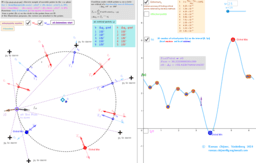### Description. Finding Geometric Medians and Geometric Centers on a circle from discrete sample points.

•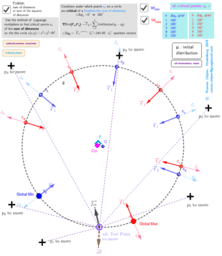### Applet. Finding Geometric Medians and Geometric Centers on a circle from discrete sample points.

•### Finding the location of geometric medians/centers on the circle from discrete sample points depending on the position of the test point.

•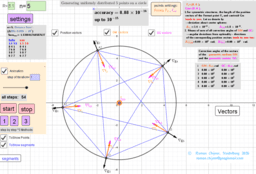### Method of Lagrange multipliers. Relative positioning of repulsive movable points on a circle.

•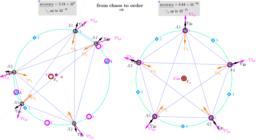### ₊Method of Lagrange multipliers. Relative positioning of "repulsive" movable points on a circle

•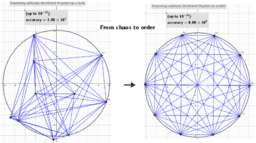### Generating an extreme arrangements of points on a circle

•### ₊Finding the optimal relative position of the" repulsive " set of particles on the circle

•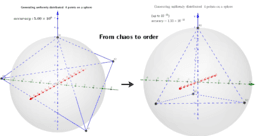### Generating an extreme arrangements of points on a sphere

•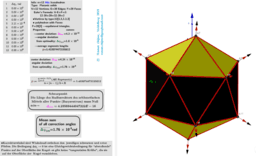### Generating an extreme arrangements of points on a sphere

•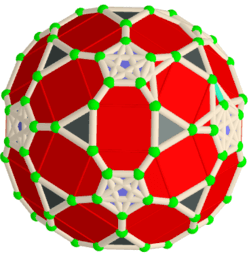### Images 1. Rhombicosidodecahedron from Biscribed Pentakis Dodecahedron for the case of trisection of its 1st-order segments

•### Images 1. Truncated icosahedron (V=60) from Biscribed Pentakis Dodecahedron for the case of trisection of its 3rd-order segments

•